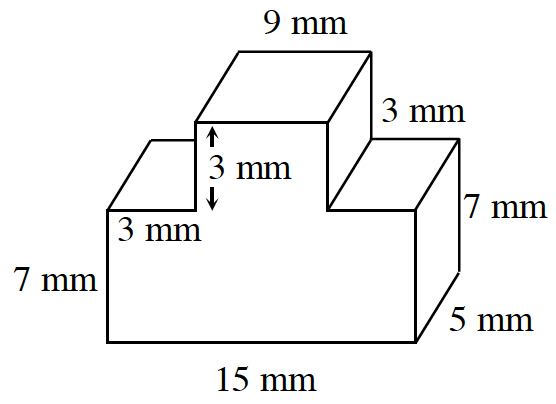### Home > CC3 > Chapter 10 > Lesson 10.1.3 > Problem10-44

10-44.

Find the volume of the prism at right. All angles are right angles.Find the area of the base (which is the side facing you) and multiply by the height of $5$ mm.

The base can be divided into two rectangles. One that is $15$ mm by $7$ mm and one that is $9$ mm by $3$ mm.

Find the area of each smaller rectangle and add them together.

$\text{Volume of a prism = (area of base)(height)}$
Now multiply the area you found for the base by the height to get the total volume of the prism.

$660 \text{ mm}^3$